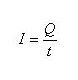# Electricity flow

The flow of electrons moving in an electrical conductor with a certain velocity called the electric current (I). The emergence of electric current because there is a potential difference at two ends of a conductor. While the potential difference in the two conductor due to one end of the conductor to get a force that drives the electrons to move.

Electric current will only occur in a closed circuit. Unit to measure the electric current is called the Ampere or abbreviated with the letter A. One coulomb charge of an electron moving through a conductor of a circuit in one second is called an Ampere. The relationship between electrical current (I), the amount of electron charge (Q), and the unit time (t) is written with the formulaWhere:
- I is the electric current (in Ampere)
- Q is the electron charge (in units of coulombs)
- T is time (in seconds)

Sample questions:
What is the size of an electric current that flows when the number of electrons of 60 coulombs in a closed circuit for half a minute?

Completion:electric currents flowing at 2 Amperes.

Next→
←Prev